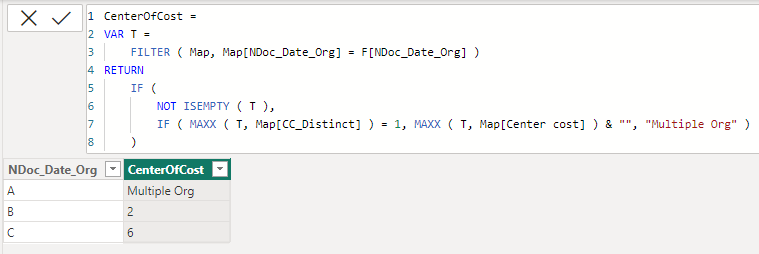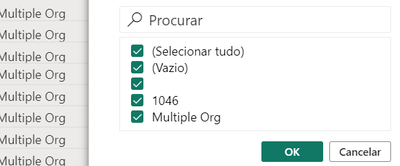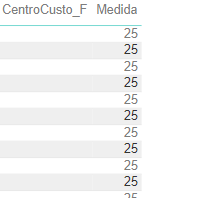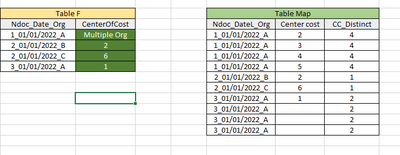cancel
Showing results for
Did you mean:Helper I

## How to make IF statements and return a result when a column from other table has equal lines

Hey, I am having some troubles here. Can you help me, please?

I made the following code to calculate a new column:

------
CenterOfCost =
IF(ISBLANK(F[NDoc_Date_Org]);BLANK();
IF(
(Map[CC_Distinct]) = 1;
LOOKUPVALUE(Map[Center cost]; Map[NDoc_DateL_Org]; F[NDoc_Date_Org])
;
IF(Map[CC_Distinct] <> 1; "Multiple Org";
BLANK())
)
)

-------

I have created some example tables for better understanding:I am not getting the right result for my CenterOfCost and i would like to get the result on my example.

Can you help?

Thanks,

Sofia

1 ACCEPTED SOLUTIONSuper User

@asofiacgraca
Please refer to attached sample file with the proposed solution``````CenterOfCost =
VAR CurrentNdocDateOrg = 'Table F'[Ndoc_Date_Org]
VAR T =
FILTER (
CALCULATETABLE (
VALUES ( 'Table Map'[Center Cost] ),
'Table Map'[NDoc_Date_Org] = CurrentNdocDateOrg
),
'Table Map'[Center Cost] <> BLANK ( )
)
RETURN
IF (
NOT ISEMPTY ( T ),
IF ( COUNTROWS ( T ) = 1, MAXX ( T, 'Table Map'[Center Cost] ) & "", "Multiple Org" )
)``````
26 REPLIES 26Super User

@asofiacgraca
Please refer to attached sample file with the proposed solution``````CenterOfCost =
VAR CurrentNdocDateOrg = 'Table F'[Ndoc_Date_Org]
VAR T =
FILTER (
CALCULATETABLE (
VALUES ( 'Table Map'[Center Cost] ),
'Table Map'[NDoc_Date_Org] = CurrentNdocDateOrg
),
'Table Map'[Center Cost] <> BLANK ( )
)
RETURN
IF (
NOT ISEMPTY ( T ),
IF ( COUNTROWS ( T ) = 1, MAXX ( T, 'Table Map'[Center Cost] ) & "", "Multiple Org" )
)``````Helper I

Hey @tamerj1

That's perfect! I really appreciate it 🙂

Best regards,

SofiaSuper User

@asofiacgraca
Here is another version that might work with the real data.``````CenterOfCost =
VAR T =
FILTER ( Map, Map[NDoc_Date_Org] = F[NDoc_Date_Org] )
RETURN
IF (
NOT ISEMPTY ( T ),
IF ( MAXX ( T, Map[CC_Distinct] ) = 1, MAXX ( T, Map[Center cost] ) & "", "Multiple Org" )
)``````Helper I

Hey

Sorry i can't provide more information since its confidential and also the volume of data a lot.

The is just returning empty spaces or 1 Center Of Cost, but that's it 😕

Also is it possible to ignore blank spaces on Map?Super User

Can you please explain further? I could not fully understand what results did you obtain. Which dax have you tried?Helper I

I tried the last code you gave me!

For example (see picture), i can have spaces that are blank and my CC_Distinct will say there are 2 values, i want it to ignore if one is a blank space, and return only the value (in the picture it would be the number 1). - last rows of the tables.Super User

Assuming Map[Center cost] is text data type, please try.

CenterOfCost =
VAR T =
FILTER (
Map,
Map[NDoc_Date_Org] = F[NDoc_Date_Org]
&& Map[NDoc_Date_Org] <> ""
)
RETURN
IF (
NOT ISEMPTY ( T ),
IF (
MAXX ( T, Map[CC_Distinct] ) = 1,
MAXX ( T, Map[Center cost] ),
"Multiple Org"
)
)

CenterOfCost =
VAR T =
FILTER (
Map,
Map[NDoc_Date_Org] = F[NDoc_Date_Org]
&& Map[NDoc_Date_Org] <> BLANK ( )
)
RETURN
IF (
NOT ISEMPTY ( T ),
IF (
MAXX ( T, Map[CC_Distinct] ) = 1,
MAXX ( T, Map[Center cost] ) & "",
"Multiple Org"
)
)Helper I

Hello,

I can't make it to work properly, it only shows one number and the rest is empty or blank:But thank you for the effort.

Best regards,

SofiaSuper User

It could be a space or a special character. Try to create a measure

LEN ( MAX ( Map[NDoc_Date_Org] ) )

and see what you getHelper I

Hey @tamerj1

I got a value of 25.Super User

I mean what value you see nex to the blank. You need to place the measure in a table visual along with the columnHelper I

That's what i getSuper User

@asofiacgraca
I don't see what you see but I thought we were talking about Map[NDoc_Date_Org] column. This CentroCusto_F column was not in the picture before and I have no idea to which table it belongs. Now I'm a bit confused which column contains the blank row? Perhaps it would be a good idea if you re-illustrate everything allover again.Helper I

Hey @tamerj1

It is the same, it's just my original file. This CentroCusto_F is the CenterOfCost I told you about and "Medida" is the measure you told me  to create now.Super User

@asofiacgraca
Ok, just create a calculated column in the same table

LEN ( Map[NDoc_Date_Org] )Helper I

I get this error due to the fact of rows with the same content, i guess: "It was not possible to determine a unique value for the "NDoc_DataLanca_Emp" column in the "MapDeviations" table."Super User

@asofiacgraca
So the NDoc_DataLanca_Emp column is the one which have the "balnk" row? Then create the column in same table.Helper I

No, it doesn't have blank values. It has the same values on multiple rows.Super User

I believe it's the time when you need to place some screenshots with proper explanation. You may hide any sensitive data and use aliases if needed.Helper I

So, i created a powerbi with the example i gave you before, for better understanding.

This is the original content and what i want to get is the CenterOfCost highlighted.As you can see below, the last row returns "Multiple Org" while it should return the number 1.Announcements#### Exclusive opportunity for Women!

Join us for a free, hands-on Microsoft workshop led by women trainers for women where you will learn how to build a Dashboard in a Day!#### Power Platform Conference-Power BI and Fabric Sessions

Join us Oct 1 - 6 in Las Vegas for the Microsoft Power Platform Conference.Top Solution Authors
Top Kudoed Authors
Users online (2,848)